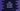# Different examples to create dart functions# Introduction :

This post will show you different examples of dart functions. Basically, the following three are the main points in a dart function :

1. arguments
2. return type
3. name

Check this post to know more about dart function :

Introduction to dart function with examples

arguments are values we are passing to a function, the return type is the return value type if the function is returning anything and name is the function name. The function name is always required because we use this name to call the function. Arguments and return types are optional.

## Example of a simple dart function :

Let’s create one function that takes one number as its argument and returns one boolean value based on the number is even or not.

``````bool checkEven(int no){
return no%2 == 0;
}``````

We can call this function from any other places of the program like :

``````main(List<string> args) {
print(checkEven(6));
print(checkEven(3));
}``````

checkEven takes one parameter, i.e. the number, it returns true if the number is even and false if it is not.

## Without using return type :

If we remove the return type from a dart function, it still works :

``````checkEven(int no){
return no%2 == 0;
}
main(List<string> args) {
print(checkEven(6));
print(checkEven(3));
}``````

It will print the same output.

## Recursive function to find out the factorial :

A recursive function is a function that calls itself to get one final result. For example, we can use one recursive function to get the factorial of a number :

``````findFactorial(int no) {
if (no == 1) return 1;
return no * findFactorial(no - 1);
}``````

We can call this function to find out the factorial of a number. If the value of that number is 1, it will return 1, else, it returns the product of that number and a recursive call to that same function with no - 1. This recursive call will again call the same function.

``````no * findFactorial(no -1)
= no * (no - 1) * findFactorial(no - 2)
= no * (no - 1) * (no - 2) * ......* 1``````

We can have a function with ‘n’ number of arguments and with a return value or without any return value.

One more type of function is available in dart called lambda, anonymous function or closure. Check the below post to learn more on it :